International
Tables for
Crystallography
Volume D
Physical properties of crystals
Edited by A. Authier

International Tables for Crystallography (2013). Vol. D, ch. 1.11, p. 270

## Section 1.11.2.1. General symmetry restrictions

V. E. Dmitrienko,a* A. Kirfelb and E. N. Ovchinnikovac

aA. V. Shubnikov Institute of Crystallography, Leninsky pr. 59, Moscow 119333, Russia,bSteinmann Institut der Universität Bonn, Poppelsdorfer Schloss, Bonn, D-53115, Germany, and cFaculty of Physics, M. V. Lomonosov Moscow State University, Leninskie Gory, Moscow 119991, Russia
Correspondence e-mail:  dmitrien@crys.ras.ru

#### 1.11.2.1. General symmetry restrictions

| top | pdf |

The most general expression for the tensor of susceptibility is exclusively restricted by the crystal symmetry, i.e.must be invariant against all the symmetry operationsof the given space group:where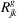is the matrix of the point operation (rotation or mirror reflection),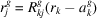, and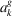is the associated vector of translation. The indexindicates a transposed matrix, and summation over repeated indices is implied hereafter. To meet the above demand, it is obviously sufficient forto be invariant against all generators of the group.

There is a simple direct method for obtainingobeying equation (1.11.2.1): we can take an arbitrary second-rank tensor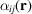and average it over all the symmetry operations: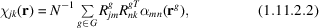whereis the number of elementsin the group. A small problem is thatis infinite for any space group, but this can be easily overcome if we takeas periodic and obeying the translation symmetry of the given Bravais lattice. Then the numberof the remaining symmetry operations becomes finite (an example of this approach is given in Section 1.11.2.3).